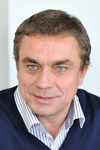Optimization: principles and algorithmsOptimization algorithms are important tools for engineers, but difficult to use. In fact, none of them is universal, and a good understanding of the different methods is necessary in order to identify the most appropriate one in the context of specific applications. Designed to teach undergraduate engineering students about optimization, this book also provides the professionals employing optimization methods with significant elements to identify the methods that are appropriate for their applications, and to understand the possible failures of certain methods on their problem. The content is meant to be formal, in the sense that the results presented are proven in detail, and all described algorithms have been implemented and tested by the author. In addition, the many numeric and graphic illustrations constitute a significant base for understanding the methods. The book features eight parts. The first part focuses on the formulation and the analysis of the optimization problem. It describes the modeling process that leads to an optimization problem, as well as the transformations of the problem into an equivalent formulation. The properties of the problem and corresponding hypotheses are discussed independently of the algorithms. Subsequently, the optimality conditions, the theoretical foundations that are essential for properly mastering the algorithms, are analyzed in detail in Part II. Before explaining the methods for unconstrained continuous optimization in Part IV, algorithms for solving systems of non linear equations, based on Newton's method, are described in Part III. The algorithms for constrained continuous optimization constitute the fifth part. Part VI addresses optimization problems based on network structures, elaborating more specifically on the shortest path problem, and the maximum flow problem. Discrete optimization problems, where the variables are constrained to take integer values, are introduced in Part VII, where both exact methods and heuristics are presented. The last part is an appendix containing the definitions and theoretical results used in the book.

Back cover, by Professor Thomas Liebling (March 2015)

Every engineer and decision scientist must have a good mastery of optimization, an essential element in their toolkit. Thus, this articulate introductory textbook will certainly be welcomed by students and practicing professionals alike. Drawing from his vast teaching experience, the author skillfully leads the reader through a rich choice of topics in a coherent, fluid and tasteful blend of models and methods anchored on the underlying mathematical notions (only prerequisites: first year calculus and linear algebra). Topics range from the classics to some of the most recent developments in smooth unconstrained and constrained optimization, like descent methods, conjugate gradients, Newton and quasi-Newton methods, linear programming and the simplex method, trust region and interior point methods. Furthermore elements of discrete and combinatorial optimization like network optimization, integer programming and heuristic local search methods are also presented.

This book presents optimization as a modeling tool that beyond supporting problem formulation plus design and implementation of efficient algorithms, also is a language suited for interdisciplinary human interaction. Readers further become aware that while the roots of mathematical optimization go back to the work of giants like Newton, Lagrange, Cauchy, Euler or Gauss, it did not become a discipline on its own until World War Two. Also that its present momentum really resulted from its symbiosis with modern computers, which made it possible to routinely solve problems with millions of variables and constraints.

With his witty, entertaining, yet precise style, Michel Bierlaire captivates his readers and awakens their desire to try out the presented material in a creative mode. One of the outstanding assets of this book is the unified, clear and concise rendering of the various algorithms, which makes them easily readable and translatable into any high level programming language. This is an addictive book that I am very pleased to recommend.

• Part I: Formulation and analysis of the problem
• Chapter 1: Formulation
• Chapter 2: Objective function
• Chapter 3: Constraints
• Chapter 4: Introduction to duality
• Part II: Optimality conditions
• Chapter 5: Unconstrained optimization
• Chapter 6: Constrained optimization
• Part III: Solving equations
• Chapter 7: Newton's method
• Chapter 8: Quasi-Newton methods
• Part IV: Unconstrained optimization
• Chapter 10: Newton's local method
• Chapter 11: Descent methods and line search
• Chapter 12: Trust region
• Chapter 13: Quasi-Newton methods
• Chapter 14: Least squares problem
• Chapter 15: Direct search methods
• Part V: Constrained optimization
• Chapter 16: The simplex method
• Chapter 17: Newton's method for constrained optimization
• Chapter 18: Interior point methods
• Chapter 19: Augmented Lagrangian method
• Chapter 20: Sequential quadratic programming
• Part VI: Networks
• Chapter 21: Introduction and definitions
• Chapter 22: The transhipment problem
• Chapter 23: Shortest paths
• Chapter 24: Maximum flow
• Part VII: Discrete optimization
• Chapter 25: Introduction to discrete optimization
• Chapter 26: Exact methods for discrete optimization
• Chapter 27: Heuristics
• Part VIII: Appendices
• Appendix A: Notations
• Appendix B: Definitions
• Appendix C: Theorems
• Appendix D: Projects
• References

AuthorBelgian, born in 1967, Michel Bierlaire holds a PhD in Mathematical Sciences from the University of Namur, Belgium. Between 1995 and 1998, he was a research associate and project manager at the Intelligent Transportation Systems Program of the Massachusetts Institute of Technology (Cambridge, Ma, USA). Between 1998 and 2006, he was a junior faculty in the Operations Research group ROSO at the Institute of Mathematics of the Ecole Polytechnique Fédérale de Lausanne (EPFL). In 2006, he was appointed associate professor at the School of Architecture, Civil and Environmental Engineering of EPFL, where he became director of the Transport and Mobility laboratory. In 2012, he was appointed full professor at EPFL. He has been teaching optimization and operations research at EPFL since 1998.